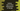# How to find Determinant in Python Numpy### How to find Determinant in Numpy:

To calculate determinant, there is one method in Numpy . We can pass a square matrix to this method and it will return the determinant value. In this post, we will learn how to use this method, its definition and few examples.

### Syntax:

Below is the syntax of the method used to calculate the determinant:

``numpy.linalg.det(arr)``

Where, arr is the square matrix, a numpy array.

It returns the determinant of the array arr. If a 2-D array is given [[x1, y1], [x2, y2]], the determinant will be x1y2 - y1x2.

### Example to find the determinant with a 2x2 matrix:

Let’s try to find the determinant of a 2x2 matrix with this method:

``````import numpy as np

arr = np.array([[4, 5], [6, 7]])

print(np.linalg.det(arr))``````

If you run this program, it will print the below output:

``-2.0000000000000013``

### Example to find the determinant for a 4x4 Numpy Matrix:

Let’s try to find the determinant for a 4x4 Numpy Matrix:

``````import numpy as np

arr = np.array([[4, 5, 6, 7], [6, 7, 8, 9], [10, 4, 1, 2], [8, 12, 3, 44]])

print(np.linalg.det(arr))``````

It will print:

``-404.00000000000125``

Similarly, we can also use this method with any other dimension matrices.

### Example to find the determinant for a stack of matrices:

We can also use the det method to find the determinant for a stack of matrices. For example,

``````import numpy as np

arr = np.array([ [[1, 21], [13, 14]], [[11, 21], [22, 21]], [[41, 53], [63, 81]] ])

print(np.linalg.det(arr))``````

It will give the determinant of all of these three matrices we passed.

``[-259. -231.  -18.]``